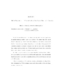## Definable families of finite Vapnik Chervonenkis dimension##### Files
umi-umd-5355.pdf(508.25 KB)
Vapnik Chervonenkis dimension is a basic combinatorial notion with applications in machine learning, stability theory, and statistics. We explore what effect model theoretic structure has on the VC dimension of formulas, considered as parameterized families of sets, with respect to long disjunctions and conjunctions. If the growth in VC dimension is linear in the number of disjunctions, then the theory under consideration has a certain kind of good structure. We have found a general class of theories in which this structure obtains, as well as situations where it fails. We relate compression schemes'' of computational learning theory to model theoretic type definitions, and explore the model theoretic implications. All stable definable families are shown to have finite compression schemes, with specific bounds in the case of NFCP theories. Notions of maximality in VC classes are discussed, and classified according to their first order properties. While maximum classes can be characterized in first-order logic, maximal classes can not